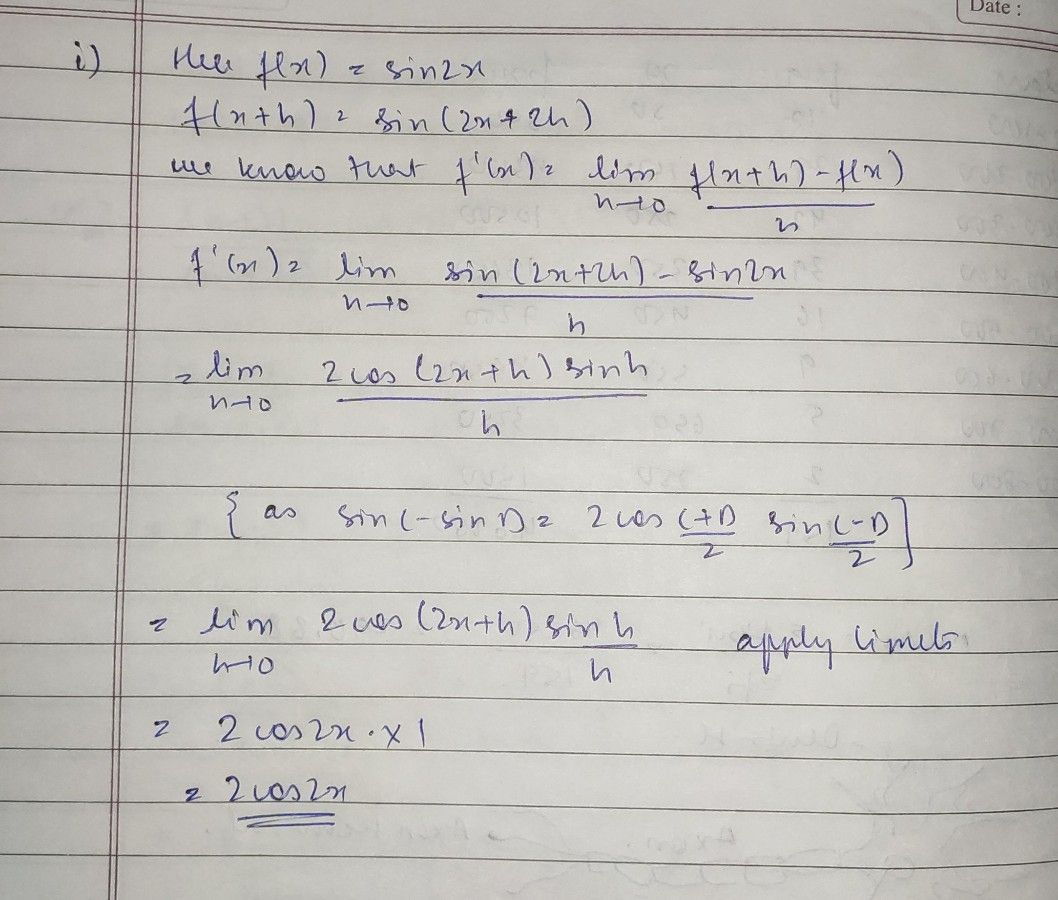Symbol
ProblemFind the derivative of the following function from the $f_{1r51}$ princlples. $sin2x$ Find the derivative of the following function from the first principles. cosax Find the derivative of the following function from the first principles. $t\square 7$ $2x$ Find the derivative of the following function from the first principle. sec3x Find the derivative of xsinx from the first principle. Prove the folle..in
10th-13th grade
Trigonometry
Search count: 135
SolutionQanda teacher - gaurav sir